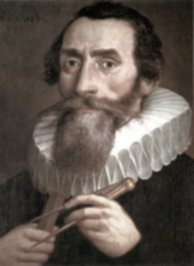Where there is matter, there is geometry.

— Johannes Kepler

## Exciting Mathematics By Mathematicians quotations

Mathematics is a more powerful instrument of knowledge than any other that has been bequeathed to us by human agency.

### The only way to learn mathematics is to do mathematics.

If only I had the Theorems! Then I should find the proofs easily enough.

Mathematics is the cheapest science. Unlike physics or chemistry, it does not require any expensive equipment. All one needs for mathematics is a pencil and paper.

Mathematics compares the most diverse phenomena and discovers the secret analogies that unite them.

Mathematics is the supreme judge; from its decisions there is no appeal.

Mathematics is the tool specially suited for dealing with abstract concepts of any kind and there is no limit to its power in this field.

I am persuaded that this method [for calculating the volume of a sphere] will be of no little service to mathematics. For I foresee that once it is understood and established, it will be used to discover other theorems which have not yet occurred to me, by other mathematicians, now living or yet unborn.

Mathematics is as much an aspect of culture as it is a collection of algorithms.

A mathematician, like a painter or a poet, is a maker of patterns.

### The product of mathematics is clarity and understanding.

Not theorems, by themselves. ... In short, mathematics only exists in a living community of mathematicians that spreads understanding and breathes life into ideas both old and new.

Since the mathematicians have invaded the theory of relativity I do not understand it myself any more.

The mathematician's patterns, like the painter's or the poet's, must be beautiful.

Logic is the hygiene the mathematician practices to keep his ideas healthy and strong.

I imagine that whenever the mind perceives a mathematical idea, it makes contact with Plato's world of mathematical concepts... When mathematicians communicate, this is made possible by each one having a direct route to truth, the consciousness of each being in a position to perceive mathematical truths directly, through the process of 'seeing'.

[Mathematics] is an independent world created out of pure intelligence.

### Mathematics is not only real, but it is the only reality.

Mathematicians are like lovers. Grant a mathematician the least principle, and he will draw from it a consequence which you must also grant him, and from this consequence another.

The union of the mathematician with the poet, fervor with measure, passion with correctness, this surely is the ideal.

### It is not certain that everything is uncertain.

It is not the job of mathematicians... to do correct arithmetical operations. It is the job of bank accountants.

Mathematics is the handwriting on the human consciousness of the very Spirit of Life itself.

### Mathematics is concerned with "all possible worlds."

I am accustomed, as a professional mathematician, to living in a sort of vacuum, surrounded by people who declare with an odd sort of pride that they are mathematically illiterate.

### I was x years old in the year x2.

A mathematician's reputation rests on the number of bad proofs he has given.

[A mathematician is a] scientist who can figure out anything except such simple things as squaring the circle and trisecting an angle.

Mathematics - the unshaken Foundation of Sciences, and the plentiful Fountain of Advantage to human affairs.

### A typical mathematician does not actively try to be useful.

Individual mathematicians are motivated primarily by a subtle mixture of ambition and intellectual curiosity, and not by a wish to benefit society, nevertheless, mathematics as a whole does benefit society.

It is the perennial youthfulness of mathematics itself which marks it off with a disconcerting immortality from the other sciences.

### Mathematics, however, is, as it were, its own explanation;

this, although it may seem hard to accept, is nevertheless true, for the recognition that a fact is so is the cause upon which we base the proof.